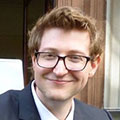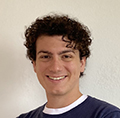# People

## FacultyBren Professor of Computing and Mathematical Sciences

Professor Stuart's research is focused on the development of foundational mathematical and algorithmic frameworks for the seamless integration of models with data. He works in the Bayesian formulation of inverse problems for differential equations, and in data assimilation for dynamical systems.

## von Karman Instructorsvon Karman Instructor in Computing and Mathematical Sciences

My research is on developing scalable computational methods for uncertainty quantification in complex physical systems. In particular, my work leverages measure transport and dimension reduction to design principled techniques for probabilistic modeling and Bayesian inference. Broadly, I am motivated by using these algorithms to improve predictions and gain insights on systems in engineering, geophysics, and medicine.von Karman Instructor in Computing and Mathematical Sciences

My research interests include partial differential equations and fluid dynamics, with practical emphases in data assimilation, optimization, high performance computing, and numerical analysis. I find the intersection of theoretical and applied mathematics to be more fruitful than studying either subject in isolation.

## Postdoctoral ScholarsFoster and Coco Stanback Postdoctoral Scholar Research Associate in Environmental Science and Engineering

Eviatar's interests lie in developing methods to improve prediction of high-dimensional, chaotic, and sparsely observed systems, with a focus on the Earth system. In particular, he has worked on methods that use tools from ensemble-based data assimilation to combine dynamical model forecasts with machine learning. He is now working on ensemble-based inversion methods to correct the statistical properties of dynamical systems. He is also interested in the application of concepts from dynamical systems theory to understand the dynamics and predictability of climate.Postdoctoral Scholar in Environmental Science and Engineering

Research Interests in Applied analysis and simulation: Partial differential equations, optimization, deterministic modelling, inverse problems, numerical analysis and implementation; Uncertainty quantification and probabilistic techniques: Bayesian inverse problems, optimal design, scalability of algorithms, machine learning.Postdoctoral Scholar in Computing and Mathematical Sciences

I am interested in the development of efficient methods for operator approximation and their application to Bayesian data assimilation for fluid flows. Part of my research focuses on emerging operator learning architectures, aiming to contribute to the theoretical underpinnings of these methods, and seeking to improve their efficiency and reliability. My other research focuses on fluid flows, their ill-posedness and implications for their reliable approximation by numerical methods.Graduate Student in Applied and Computational Mathematics

I come from a numerical PDEs background, having worked on finite difference, finite element and pseudo-spectral methods for Schroedinger and Navier--Stokes equations. My current research is developing data assimilation tools for global climate models, in particular, the focus is on experimental design questions and ODE/PDE averaging. I am also interested in Koopman analysis, diffusion maps, and parameter estimation.Graduate Student in Applied and Computational Mathematics

My work lies at the intersection of data assimilation, stochastic analysis, dynamical systems, computational statistics and machine learning. In general, I am interested in using theoretical insights from analysis to develop novel computationally efficient numerical algorithms.My research delves into the area of high-dimensional chaotic dynamical systems. I am particularly interested in extreme events, data assimilation, uncertainty quantification, and the application of machine learning to uncover constitutive laws. Currently, my focus is on studying the predictability of earthquakes and Slow Slip Events (SSEs). In the intersection of mathematics, geophysics, and data science, my work aims to shed light on complex, high-dimensional systems that have the potential to improve our understanding and ability to predict natural phenomena.Graduate Student in Environmental Science and Engineering

Ervik’s research interests broadly encompass stochastic models for the climate. He has a background in applied mathematics and is currently working on developing stochastic closures for subgrid-scale cloud models. The goal of this research is to develop a model that more faithfully incorporates uncertainty. As part of this, he also works on methods for robust parameter estimation and uncertainty quantification.Graduate Student in Applied and Computational Mathematics and Mechanical Engineering

I have research interests in theory and algorithms for high-dimensional scientific and data-driven computation. My current work is centered on operator regression, with application to efficient surrogates for forward and inverse problems arising from models of physical systems. To this end, I develop and utilize tools from machine learning, model reduction, and numerical/statistical analysis.Graduate Student in Applied and Computational Mathematics

I am interested in problems at the intersection of mechanics, data-driven modelling, statistics and machine learning. Currently, I am working on multiscale modelling problems and learning thermodynamically stable material constitutive laws from data.